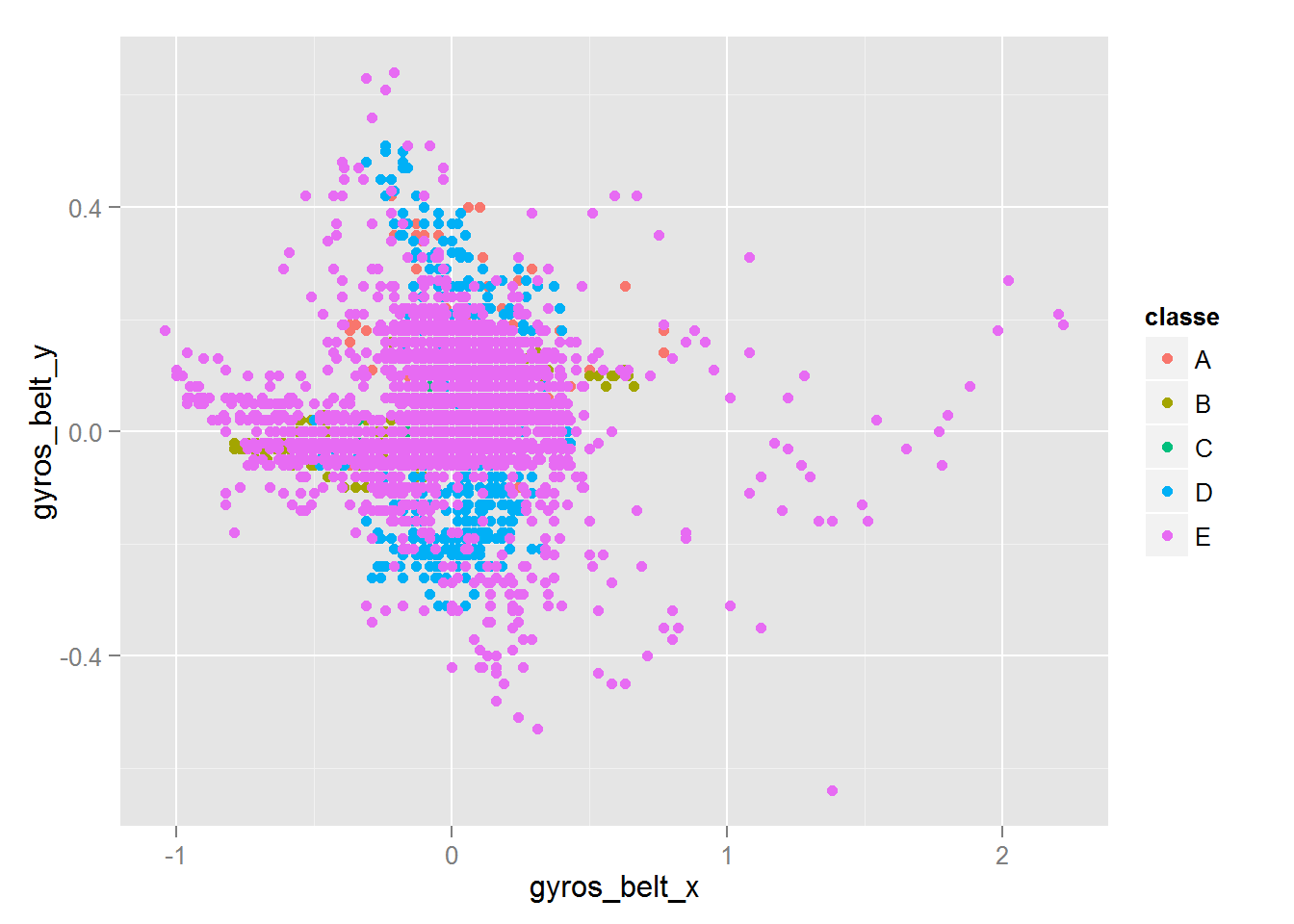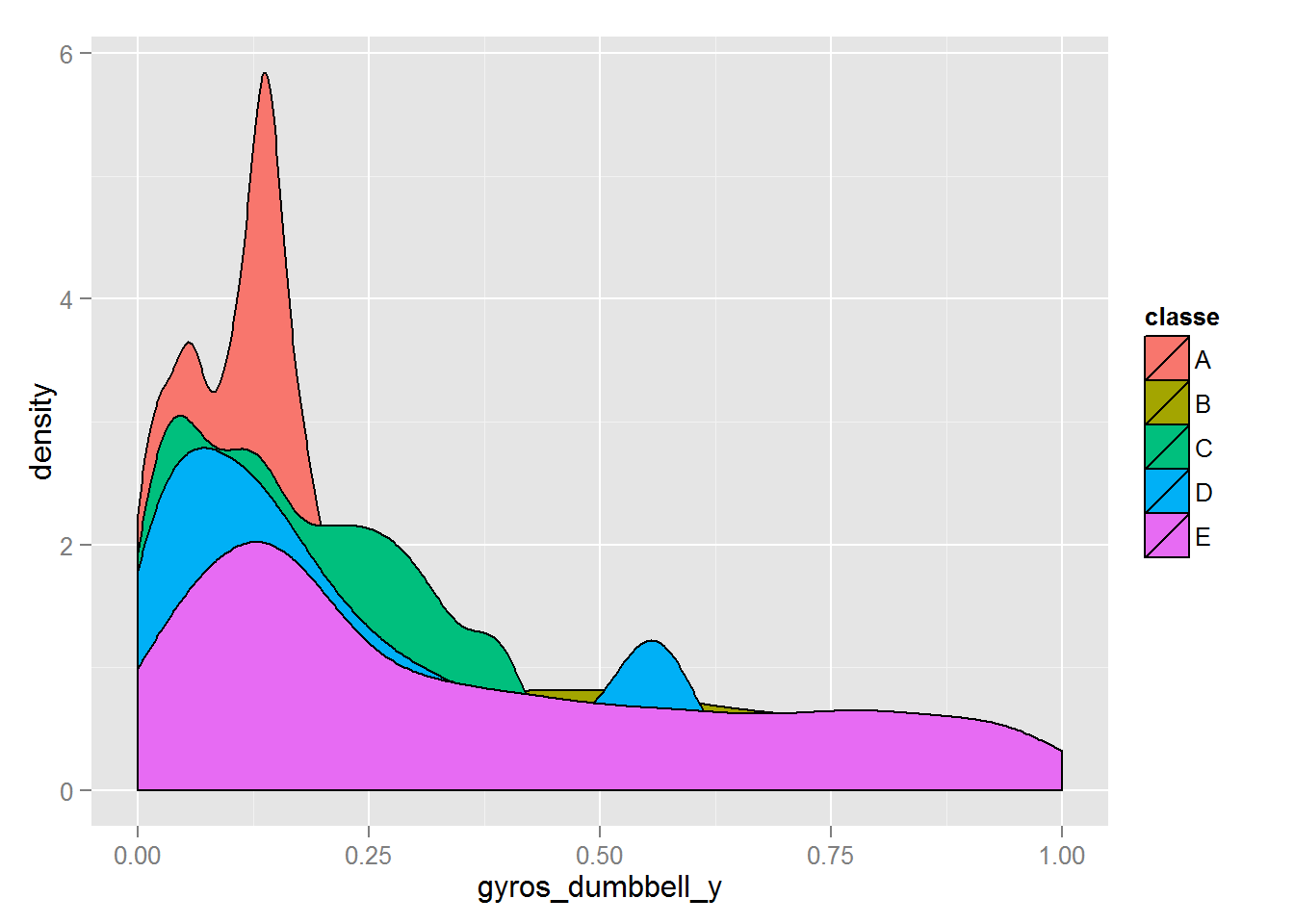# Load and prepare the data

data <- read.csv("pml-training.csv")
dim(data)
##  19622   160
table(data$classe) #manner to be predicted ## ## A B C D E ## 5580 3797 3422 3216 3607 Using summary(data), learning about variables. Some variables with too many NAs or Blank, and first 7 variables about the number(X), user, time and windows, are also irrelevant. Removing these variables from predictors. v <- apply(is.na(data)|data=="", 2, sum) sum(v==0); sum(v>19000) ##  60 ##  100 data <- subset(data[,v==0]); data <- data[-c(1:7)] dim(data) ##  19622 53 # Explore the data Now we have 53 variables, combination of: 6 different ways: roll, pitch, yaw, gyros, accel and magnet 4 accelerometer locations: belt, arm, dumbbell, forearm Now exploring variables with some plots.# Machine learning ## Split data to training & testing sets for cross validation set.seed(23123) library(caret) inTrain <- createDataPartition(y=data$classe, p=0.6, list=FALSE)
train <- data[inTrain,]; test <- data[-inTrain,]

## Classification Model

It’s a question of classification.

Trying to use classification tree, random forest, model based prediction nad moosting. With default setting, method=“lda” is best for its accuracy and speed.

The Accuracy is about 0.70, so the out of sample error is expected 0.30.

modFit <- train(classe~., method="lda", data=train)
modFit$result ## parameter Accuracy Kappa AccuracySD KappaSD ## 1 none 0.6969599 0.6164363 0.007214281 0.009004911 pred <- predict(modFit, test) table(pred, test$classe)
##
## pred    A    B    C    D    E
##    A 1838  259  141   90   61
##    B   40  973  123   62  238
##    C  195  159  902  149  144
##    D  153   55  160  944  149
##    E    6   72   42   41  850
sum(pred==test\$classe)/dim(test) # Testing Set's Accuracy
##  0.7018863

The accuracy of testing set is a little better than that of training set.

It’s not so good. But is as expected.

# Machine learning algorithm apply to Test data

For submission of predictions, use the model to original Test data.

testing <- read.csv("pml-testing.csv")
answer <- predict(modFit, testing); answer
##   B A B C C E D D A A D A B A E A A B B B
## Levels: A B C D E